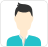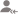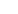true

Find the best tutors and institutes for MBA Entrance Coaching

Find Best MBA Entrance Coaching classes

No matching category found.

No matching Locality found.

Outside India?

Search for topicsPost a Lesson

All

All

Lessons

Discussion

Lesson Posted on 14/10/2019 Exam Coaching/MBA Entrance CoachingShantanav Bhowmik

My recent academic accomplishments include admission offers (unreserved category) from MDI Gurgaon and...

SB Page 1PROBABILITY- STUDY NOTESPROBABILITY- the method of finding the likelihood of an event.1. For example let us throw a dice,an unbiased dice that is all faces have equal chances of turning up -1,2,3,4,5,6 on six faces. What is the probability that the number up will be an even number?sample space=1,2,3,4,5,6=>... read more

SB Page 1
PROBABILITY- STUDY NOTES
PROBABILITY- the method of finding the likelihood of an event.
1. For example let us throw a dice,an unbiased dice that is all faces have equal chances of turning up -
1,2,3,4,5,6 on six faces. What is the probability that the number up will be an even number?
sample space=1,2,3,4,5,6=> n(s)=6
even number or event space= 2,4,6=> n(e)=3
n()=number of elements
therefore required probability= n(e)/n(s)=3/6=1/2.
P(E)=N(E)/N(S)
2. Now unbiased 2 dies are thrown simultaneously. What is the probability that sum of their faces is 8?
we know that the minimum value on each die is 1 and maximum value is 6.
we also know that we can get a sum of 8 with integers from 1 to 6 as=3+5/4+4
so the number of ways in which the required sum of 8 can face up is=
Dice1 Dice2
4 4
5 3
3 5
so the required number of cases for or event= 4/4,3/5,5/3= 3 cases.
total number of cases (from method of counting)=6*6=36
P(E)=3/36=1/12
2nd method- what is the probability that 4 will turn up in die 1? It is 1/6 as n(e)=1 & n(s)=6. Similarly for
every other number on an unbiased die.
A sum of 8 will be obtained when we get –{(4 on d1) &( 4 on d2)} OR{ (3 on d1) &( 5 on d2)} OR {(5 on d1)
& (3 on d2)} as listed above.
Therefore the required probability is obtained when we replace & by X (multiplication) and OR by +
(addition). And we write the probability for every individual event in the bracket terms.
so P(e)= { 1/6*1/6}+{1/6*1/6}+{1/6*1/6)=1/12.
3. Consider again an unbiased die .
n(even)=3 since cases{2,4,6)
n(odd)=3 since cases (1,3,5)
As set of cases of odd number and set of cases of even number has no element common even if their number
of elements is same –these two sets are said to be mutually exclusive.
Hence we say that the two events of getting odd numbers and even numbers when an unbiased dice is
thrown is mutually exclusive to each other.
4. N(AUB)=N(A)+N(B)-N(A˄B) from SET theory : S = sample space ,in this case represents all possible
outcomes- the whole box.
here if we are asked to find the probability that either A or B occurs ( it means atleast one of A or B) , we will
take help of the above concept of vein diagram as given above.
P(e)= n(A∪B)/n(s)
A A˄B B
S
SB Page 2
5. Consider the case of an unbiased die again.
Let A be the case of getting a prime ∴ N(A)=(2,3,5)=3 ∴P(A)=N(A)/N(S)=3/6=1/2.
Let B be the case of getting an even no ∴ N(B)=(2,4,6)=3 ∴P(B)=N(B)/N(S)=3/6=1/2.
What if we are asked to find out the probability of event A in case it is given that event B has already
occurred?
In this case we find the the outcomes of B are the that is n(b)=3. In these outcomes we are to find the prime
numbers that are in set A to get our event space. So our event space n(e)=n(A˄B)={2}=1 and our sample
space n(b)=3.
So the req P(e)= N(A˄B)/N(B)= 1/3.
This is written as P(A/B)- probability that A will occur given B has already occurred.
Now as req event space is A˄B; We write P(A/B)=P(A˄B)/P(B). This known as conditional probability.
This can be derived as follows- We know P (A/B)=N(A˄B)/N(B)= *N(A˄B)N(S)+/*N(B)/N(S)+=
P(A/B)=P(A˄B)/P(B).
6. Consider set S =*1,2,3,4………47,48,…..50+
A= Event of getting a multiple of 5 from S= { 5,10,15,……,50+
B= Event of getting a multiple of 2 from S =*2,4,6,8………..50+
now P(A)=10/50 , P(A/B)=5/25
As we see that numerically P(A)=P(A/B) that is the probability of event A has not changed given the
condition that B has already occurred , we say that A & B are independent events. As we know that
P(A/B)=P(A˄B)/P(B). Putting the condition for independent events that is P(A)=P(A/B) we get
P(A).P(B)=P(A˄B)
As A & B are independent events as proven we can also show P(A/ )=P(A).
N:B- If P(A˄B)=P(A).P(B) numerically, A &B are pairwise independent.
Similarly if P(A˄B˄C)=P(A).P(B).P(C) , A,B & C are mutually independent.
7. BAYES THEOREM of Conditional Probability
If we are given the probabilities that B occurs given A1, A2…..An occurs and we are asked to find the
probability that Ai will occur given B has already occurred, when we are given or can calculate P(Ai), we use
Bayes theorem.
Note that A1 A2 A3 …..An are mutually exclusive and collectively exhaustive that is individual event spaces
don’t have any common and all As make up A event space.
Given, Mathematically if P(B/Ai)=Qi and P(Ai)=Pi then
P(Ai/B)= Pi.Qi/𝞢Pi.Qi
Example-
Box 1 contains 3 white and 2 black balls. Box 2 contains 1 white and 4 black balls.A ball is picked from one
of the two boxes, it turns out to be black. Find the probability that it was drawn from box 1.
We are to find probability of selecting box 1 given we have picked a black ball=P(B1/BLACK)
By BAYES THEOREM,
P(B1/BLACK)={P(B1).P(BLACK/B1)}/[{ P(B1).(BLACK/B1)}+ {P(B2).P(BLACK/B2)}]
Hence,
P(BLACK/B1)=Probability of drawing a black ball from box 1= 2/5
P(BLACK/B2)=Probability of drawing a black ball from box 2= 4/5
P(B1)=1/2
P(B2)=1/2
∴ P(B1/BLACK)= (1/2)(2/5)/,*(1/2)(2/5)++*(1/2)(4/5)+-=1/3
™Solution of Bayes theorem problem without Bayes theorem
Consider the given problem above.
Note that since we are given that a black ball has been picked already that reduces out sample space
probability to selecting bag 1 and black ball or selecting bag 2 and black ball while the former is our event
SB Page 3
space probability. So basically it reduces to the probability of the way or ways we can get the desired
outcome or outcomes divided by the summation of all the probabilities of available outcomes , given the pre
condition.
Probability of selecting box 1 given we have picked a black ball=
P(B1/BLACK)= event space probability P(e)/ sample space probability P(s) = event of selecting black ball
from B1/ events of selecting black balls from B1 & B2=event of selecting B1 and black ball from it/[ event of
selecting B1 and black ball from it OR event of selecting B2 and black ball from it]= 1/3
8. Expected Value= E1.M1+E2.M2+…….+En.Mn
Example-
A person tosses a coin. It comes up with head he gets 10 rupees. It comes up with tail he has to pay 5 rupees.
What is his expectation ?

Dislike Bookmark

Lesson Posted on 08/10/2019Rahul Ranjan

I have worked in various MBA/BANKING preparation institutes across India. Expert in Concept building...

Dislike BookmarkCareer Desk

Dear Sambit, Yes, MAT exam can be easily cracked. However, for CAT, it is difficult but not impossible. Plan and strategize and implement. If you are focussing full time for the next three months and practice smartly. You will definetely achieve good percentile. Wishing you all the best.
Dislike Bookmark

Looking for MBA Entrance Coaching classes

Find best MBA Entrance Coaching classes in your locality on UrbanPro.

FIND NOW

Lesson Posted on 25/07/2019Anubhav

Suppose we want to find highest power of a number(n) in n factorial m It is calculated by =++.... where is greatest integer Example if we want to find highest power of 5 in 30 factorial It will be + =6+1 So highest power of 5 in 30 factorial is 7 read more

Suppose we want to find highest power of a number(n) in n factorial m

It is calculated by

=[m/n]+[m/n*n]+[m/n*n*n]....

where [] is greatest integer

Example if we want to find highest power of 5 in 30 factorial

It will be [30/5]+[30/25]

=6+1

So highest power of 5 in 30 factorial is 7

Dislike Bookmark

Lesson Posted on 24/07/2019 Exam Coaching/MBA Entrance CoachingAnubhav

If a number a + b + c is divided by number n then reminder will be sum of reminder if each one is divided by n Example 3 + 4 + 9 divide by 12 what will be reminder 3+4-3=4 will be the reminder here we have used the concept of negative reminder read more

If a number a + b + c is divided by number n then reminder will be sum of reminder if each one is divided by n

Example

3 + 4 + 9 divide by 12 what will be reminder

3+4-3=4 will be the reminder here we have used the concept of negative reminder

Dislike BookmarkAtul Mittal

Most Qualified MBA Home Tutor Teaching in TIME CP from 13 Yrs

Forget about profile. Just try getting as high a percentile as possible in CAT. Written +GD/PI/WAT form the major chunk of the selection process in any top b school.
Dislike Bookmark

When should I Start my CAT preparations?Ajay Dhar

Guarantee of better results than most, or FREE Tutoring for Entrance Competitions, IIT, NEET, CAT

if you start one year ago, you need less efforts. one hour a day is sufficient. if you start late, 10 hours a day may be needed. I give one hour coaching for free.
Dislike Bookmark

Answered on 19/07/2019 Exam Coaching/MBA Entrance CoachingSrinjini A.

Hi Jennifer, please clear your basics first. Get in touch with someone who offers courses on the basics and clears your concepts. Once that is made, your path is clear. I would suggest you start with a lower level of difficulty. I have used Arun Sharma for my preparations and its time consuming but... read more

Hi Jennifer, please clear your basics first. Get in touch with someone who offers courses on the basics and clears your concepts. Once that is made, your path is clear. I would suggest you start with a lower level of difficulty.

I have used Arun Sharma for my preparations and its time consuming but useful. I hold an MBA myself, and I can say you will require patience in your preps. All the Best :) I am open to all kinds of necessary suggestions.

Dislike Bookmark

Answered on 08/06/2018 Exam Coaching/MBA Entrance Coaching Tuition/BBA TuitionAtul Mittal

Most Qualified MBA Home Tutor Teaching in TIME CP from 13 Yrs

Dislike Bookmark

Looking for MBA Entrance Coaching classes

Find best MBA Entrance Coaching classes in your locality on UrbanPro.

FIND NOWDislike Bookmark

UrbanPro.com helps you to connect with the best MBA Entrance Coaching classes in India. Post Your Requirement today and get connected.

Overview

Questions 1.1 k

Lessons 43

Total Shares31,681 Followers

## MBA Entrance Coaching in:

x

X

### Looking for MBA Entrance Coaching Classes?

Find best tutors for MBA Entrance Coaching Classes by posting a requirement.

• Post a learning requirement
• Get customized responses
• Compare and select the best### Looking for MBA Entrance Coaching Classes?

Find best MBA Entrance Coaching Classes in your locality on UrbanProUrbanPro.com is India's largest network of most trusted tutors and institutes. Over 25 lakh students rely on UrbanPro.com, to fulfill their learning requirements across 1,000+ categories. Using UrbanPro.com, parents, and students can compare multiple Tutors and Institutes and choose the one that best suits their requirements. More than 6.5 lakh verified Tutors and Institutes are helping millions of students every day and growing their tutoring business on UrbanPro.com. Whether you are looking for a tutor to learn mathematics, a German language trainer to brush up your German language skills or an institute to upgrade your IT skills, we have got the best selection of Tutors and Training Institutes for you. Read more